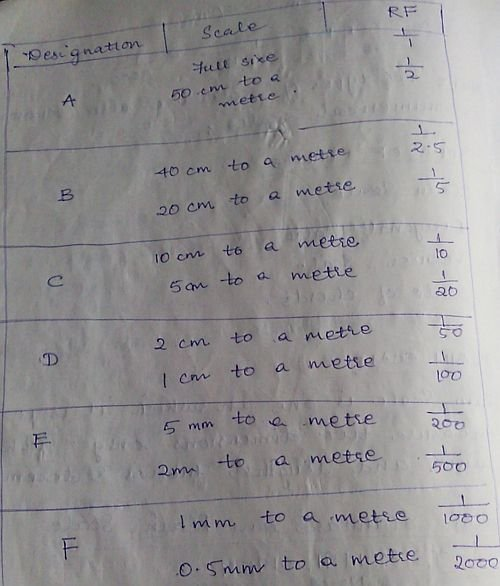## Sources of Errors in Surveying | Civil Engineering

#### Sources of Errors in Surveying

In this article, we will discuss three major types of errors that are found to be very common in Surveying.Types of Errors

#### Types of Errors

1. Instrumental errors
2. Personal errors
3. Natural errors

#### Instrumental errors

Error may arise due to imperfection or faulty adjustment of the instrument with which measurement is being taken.

For example:

A tape may be too long or an angle measuring instrument maybe out of adjustment. Such errors are known as Instrumental erros.

Read more

## Types of Scales in Engineering Surveying

#### Types of Scales | Engineering Surveying

In the previous article, we discussed briefly on the topic of “Scales in Surveying” where we came across an important term “Representative factor” which forms an important part in understanding the scales in Surveying.

In this article, we will discuss different types of scales used in Surveying…

#### The scales are classified into four categories:

1. Plain Scale
2. Diagonal Scale
3. Vernier Scale
4. Scale of chords

Lets go on with the discussion of types of scales briefly for our better understanding…

#### Plain Scale

Plain Scale is one on which it is possible to measure two dimensions only. For example, measurements such as units and lengths, metres and decimetres etc.Plain Scale

Six different plain scales in metric used by engineers, Architects and Surveyors.

#### Diagonal Scale

On diagonal scale, it is possible to measure three dimensions such as metres, decimetres and centimetres, units , tens and hundreds; yards, feet and inches etc.

A short length is divided into number of parts using the principle of similar triangle in which sides are proportional.

Read more

## Methods of Calculation of Areas in Surveying | Simpson’s Rule

#### Calculation of Areas in Surveying | Simpson’s Rule

In one of my previous articles, I discussed Midpoint Ordinate Rule and Average Ordinate Rule in detail with an example and listed out various important methods used for the calculation of areas in Surveying. In this article, we will deal with the next important method (rule) i.e. Simpson’s Rule along with a numerical example used for the calculation of areas in the field of Surveying.

#### Here are the five important rules (Methods) used for the calculation of areas in Surveying:

1. Midpoint ordinate rule
2. Average ordinate rule
3. Simpson’s rule
4. Trapezoidal rule
5. Graphical rule

#### Simpson’s Rule

Statement

It states that, sum of first and last ordinates has to be done. Add twice the sum of remaining odd ordinates and four times the sum of remaining even ordinates. Multiply to this total sum by 1/3rd of the common distance between the ordinates which gives the required area.

Where O1, O2, O3, …. On are the lengths of the ordinates

d = common distance

n = number of divisions

#### Note:

This rule is applicable only if ordinates are odd, i.e. even number of divisions.

If the number of ordinates are even, the area of last division maybe calculated separated and added to the result obtained by applying Simpson’s rule to two remaining ordinates.

Read more

## Contour Analysis | Guide to Surveying and Levelling

#### Importance of Contouring in the field of Surveying

Contouring is an imaginary line on the ground obtained by joining points having same elevation.

#### Characteristics of Contours

Contour lines are closed, however they may be close on the map itself or outside the map depending upon the topography.

The spacing between contour lines depends upon the slope of the ground.

In steep slopes, the spacing is small, for gentle slopes the spacing is large.

If the contour lines are equally spaced, they indicate uniform slope.Contour Analysis

If the contour lines are parallel, straight or equally placed, they represent plane surface.

In a series of contour lines on the plan or map indicates either a hill or depression.

In case of the hill, the values of the elevation go on increasing towards the centre whereas in case of depression, the values go on decreasing towards the centre.

Read more